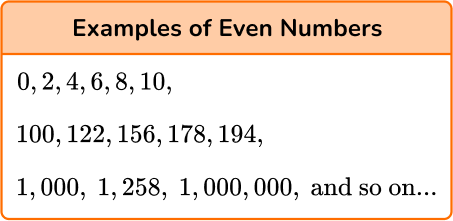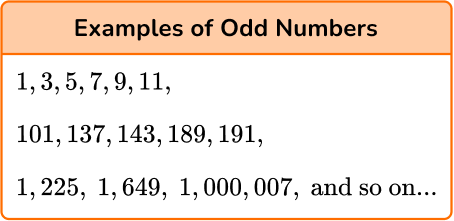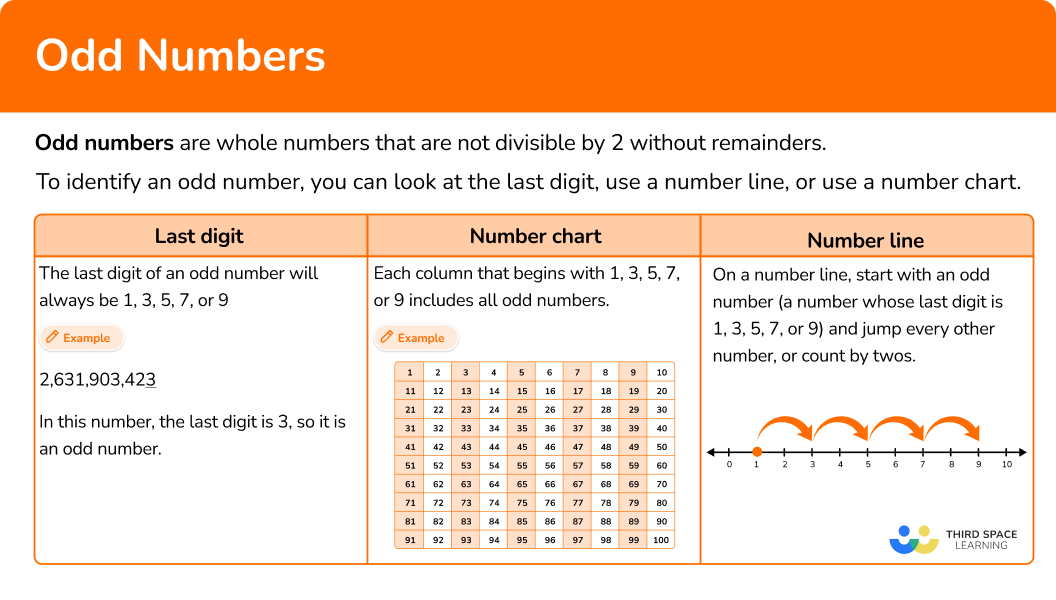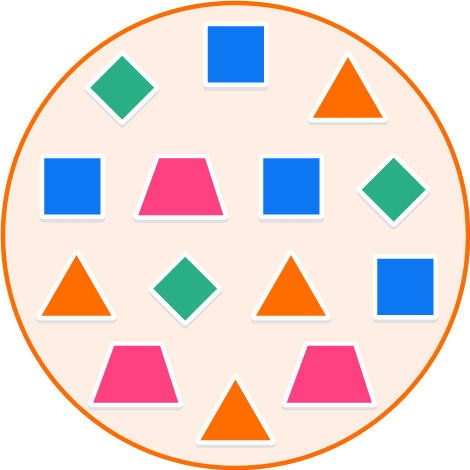# Odd numbers

Here you will learn about odd numbers, including examples of odd numbers, odd numbers on a number line and a number chart, and properties of odd numbers.

Students will first learn about odd numbers as part of operations and algebraic thinking in 2nd grade. They expand upon their knowledge of odd numbers in 3rd grade when they identify arithmetic patterns and properties of numbers.

## What are odd numbers?

Odd numbers are whole numbers that are not divisible by 2 without remainders.

The last digit of an odd number is 1, 3, 5, 7, or 9.

Odd numbers are the opposite of even numbers, which are divisible by 2. An even number’s last digit is 0, 2, 4, 6, or 8.

For exampleTo identify an odd number, you can look at the last digit, use a number line, or use a number chart.

Last digit

The last digit of a number, or the digit in the ones place, will tell
you if it is even or odd.

The last digit of an odd number will always be
\bf{1, 3, 5, 7,} or \bf{9} .

2,631,903,42\underline{3}

In this number, the last digit is 3, so it is an odd number.

Number line

Start with a number whose last digit is 1, 3, 5, 7, or 9 and jump
every other number, or count by twos.

Number chart

On a number chart each column that begins with 1, 3, 5, 7, or 9
includes odd numbers.

This chart shows a list of odd numbers between 1 and 100.

Properties of even numbers and odd numbers

• If you add an even number to an even number, the sum will always be an even number. For example, 8 + 4 = 12 .
• If you add an even number to an odd number, the sum will always be an odd number. For example, 8 + 3 = 11 .
• If you add an odd number to an odd number, the sum will always be an even number. For example, 5 + 3 = 8 .

• Property of subtraction
• If you subtract an even number from an even number, the difference will always be an even number. For example, 16-10 = 6 .
• If you subtract an even number from an odd number or an odd number from an even number, the difference will always be an odd number. For example, 16-9 = 7; 17-10 = 7 .
• If you subtract an odd number from an odd number, the difference will always be an even number. For example, 9-5 = 4 .

• Property of multiplication
• If you multiply an even number by an even number, the product will always be an even number. For example, 4 \times 2 = 8.
• If you multiply an even number by an odd number, the product will always be an even number. For example, 4 \times 5 = 20 .
• If you multiply an odd number by an odd number, the product will always be an odd number. For example, 3 \times 5 = 15 .

Groups of objects

• To determine if a group of objects has an even number of objects or an odd number of objects, group the objects into pairs, or equal groups of 2. If each object can be grouped into a pair, there is an even number of objects. If there is one object left over after pairing, there is an odd number of objects.

For example,

Since all of the objects in this group can be
grouped into pairs, there is an even number
of objects.

After grouping the objects into pairs, there is
one left over. Therefore, there is an odd number
of objects in this group.

### What are odd numbers?## Common Core State Standards

How does this relate to 2nd grade math?

• Grade 2 – Operations and Algebraic Thinking (2.OA.3)
Determine whether a group of objects (up to 20 ) has an odd or even number of members, for example, by pairing objects or counting them by 2 s; write an equation to express an even number as a sum of two equal addends.

## How to identify odd numbers

In order to identify odd numbers:

1. Look at the last digit, or the digit in the ones place. If the digit is \bf{1, 3, 5, 7,} or \bf{9,} the number is odd.
2. Use this strategy to answer the question.

In order to determine if the answer to an equation will be an odd number:

1. Recall the properties of addition, subtraction, or multiplication for odd numbers.
2. Apply the correct property.

In order to determine if there is an odd number of objects in a group:

1. Group the objects into pairs.
2. If all objects can be grouped into pairs, there is an even number of objects. If there is one left over, there is an odd number of objects.

## Odd numbers examples

### Example 1: identifying odd numbers

True or false: 823 is an odd number.

1. Look at the last digit, or the digit in the ones place. If the digit is \bf{1, 3, 5, 7,} or \bf{9,} the number is odd.

The last digit, or the digit in the ones place, is 3.

2Use this strategy to answer the question.

Since the last digit in 823 is 3, it is an odd number, so the statement is true.

### Example 2: identifying odd numbers

List all consecutive odd numbers between 61 and 71.

Look at the last digit, or the digit in the ones place. If the digit is \bf{1, 3, 5, 7,} or \bf{9,} the number is odd.

Use this strategy to answer the question.

### Example 3: identifying odd numbers

Look at the list of numbers. Which numbers are odd?

17, 438, 282, 500, 901, 744, 355, 483, 809, 620

Look at the last digit, or the digit in the ones place. If the digit is \bf{1, 3, 5, 7,} or \bf{9,} the number is odd.

Use this strategy to answer the question.

### Example 4: apply properties of odd numbers

Joe is subtracting an odd number from an odd number. He thinks the difference will be an odd number, but his friend Samira says it will be even. Who is correct?

Recall the properties of addition, subtraction, or multiplication for odd numbers.

Apply the correct property.

### Example 5: apply properties of odd numbers

Anna solves the following equation:

105,915 + 27,863 = 133,775

Her friend glances at the equation and tells her it’s incorrect. How does her friend know this is incorrect?

Recall the properties of addition, subtraction, or multiplication for even numbers.

Apply the correct property.

### Example 6: determine if a group of objects is odd

Look at the objects in the square. Is there an odd number of objects?

Group the objects into pairs.

If all objects can be grouped into pairs, there is an even number of objects. If there is one left over, there is an odd number of objects.

### Teaching tips for odd numbers

• Hang a number chart, or hundreds chart, in your classroom with odd numbers highlighted.

• Provide worksheets with practice questions that require students to find odd numbers between any given numbers. For example, instead of always starting at 0, give them a starting number of 63 and ask them to find the odd numbers through 100.

• Provide worksheets with practice questions that ask students to find the next odd numbers and find consecutive odd numbers to build on their understanding of odd numbers. Allow students to use a number line if needed.

### Easy mistakes to make

• Thinking you can’t count by \bf{2} s using odd numbers
Students may think that you can only count by 2 s using even numbers since odd numbers are not multiples of 2. However, if you start at an odd digit and count by 2 s, each number will be odd.

• Thinking that fractions and decimals can be odd numbers
Only integers (whole numbers and their corresponding negative numbers) can be odd numbers. Students may think that a number such as 2.43 is an odd number because its last digit is a 3. However, this is not the case as fractions and decimals can not be even numbers or odd numbers.

### Practice odd numbers questions

1. Which of the following is an odd number?

15805221The last digit of an odd number is 1, 3, 5, 7, or 9.

Therefore, 21 is an odd number.

2. What is the smallest odd number?

0210.1One is the smallest odd number because it is the smallest positive whole number that is not divisible by 2.

3. What are the odd numbers between 195 and 204?

195, 196, 197, 198, 199, 200, 201, 202, 203, 204196, 198, 200, 202, 204195, 197, 199, 200, 201, 203195, 197, 199, 201, 203The last digit of an odd number is always 1, 3, 5, 7, or 9.

195, 197, 199, 201, and 203 are the only set of numbers that all end in one of those digits.

4. When you add an odd number and an odd number, the answer will…

always be an even number.sometimes be an even number.never be an even number.always be an odd numberThe property of addition of odd numbers says that when you add an odd number to an odd number, the sum will always be an even number.

5. True or false: When you subtract an odd number from an odd number, you will get an odd number.

True because the property of subtraction of odd numbers says \text{odd number } – \text{ odd number } = \text{ odd number}False because the property of subtraction of odd numbers says \text{odd number } – \text{ odd number } = \text{ even number}True because the property of subtraction of odd numbers says \text{odd number } – \text{ odd number } = \text{ even number}False because the property of subtraction of odd numbers says \text{odd number } – \text{ odd number } = \text{ odd number}The property of subtraction of odd numbers says when you subtract an odd number from an odd number, the difference will always be an even number.

6. Look at the group of shapes inside the circle. Is there an odd number of shapes?Yes because if you group the shapes into pairs, there is one left over.No because if you group the shapes into pairs, there is one left over.No because if you group the shapes into pairs, there are none left over.Yes because if you group the shapes into pairs, there are none left over.If you group the shapes into pairs, you will see there are none left over.

This means there is an even number of shapes. (The total number of shapes is 14. )

## Odd numbers FAQs

What is an odd number?

An odd number is a whole number that is not a multiple of 2. This means that odd numbers are not divisible by 2 with no remainders.

What is the difference between an even number and an odd number?

An even number is divisible by 2 with no remainders and an odd number is not. The last digit of an even number is 0, 2, 4, 6, or 8, while the last digit of an odd number is 1, 3, 5, 7, or 9.

Can fractions be odd numbers?

Even numbers and odd numbers are all positive integers and negative integers. Therefore, fractions and decimals are neither even nor odd.

## Still stuck?

At Third Space Learning, we specialize in helping teachers and school leaders to provide personalized math support for more of their students through high-quality, online one-on-one math tutoring delivered by subject experts.

Each week, our tutors support thousands of students who are at risk of not meeting their grade-level expectations, and help accelerate their progress and boost their confidence.